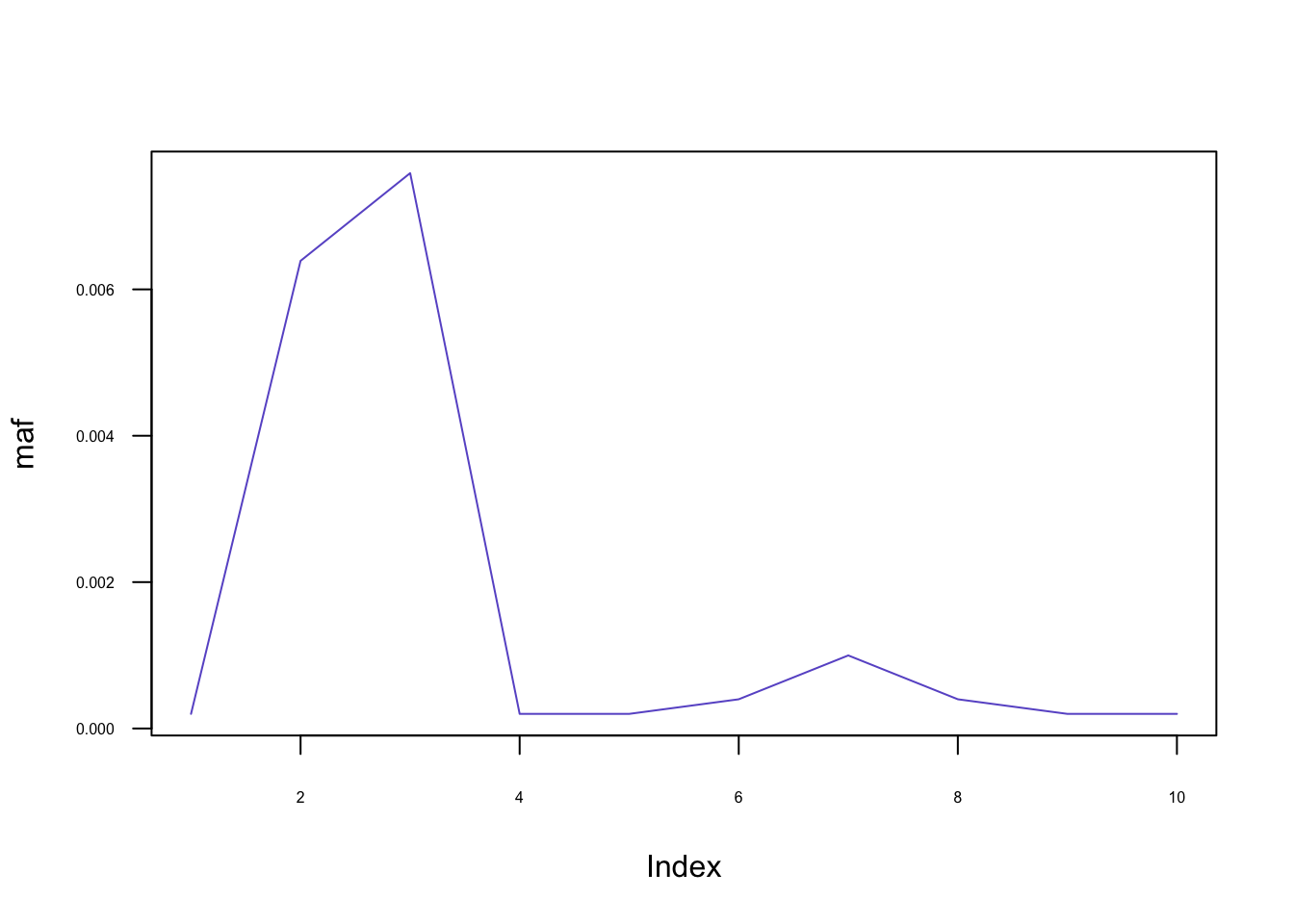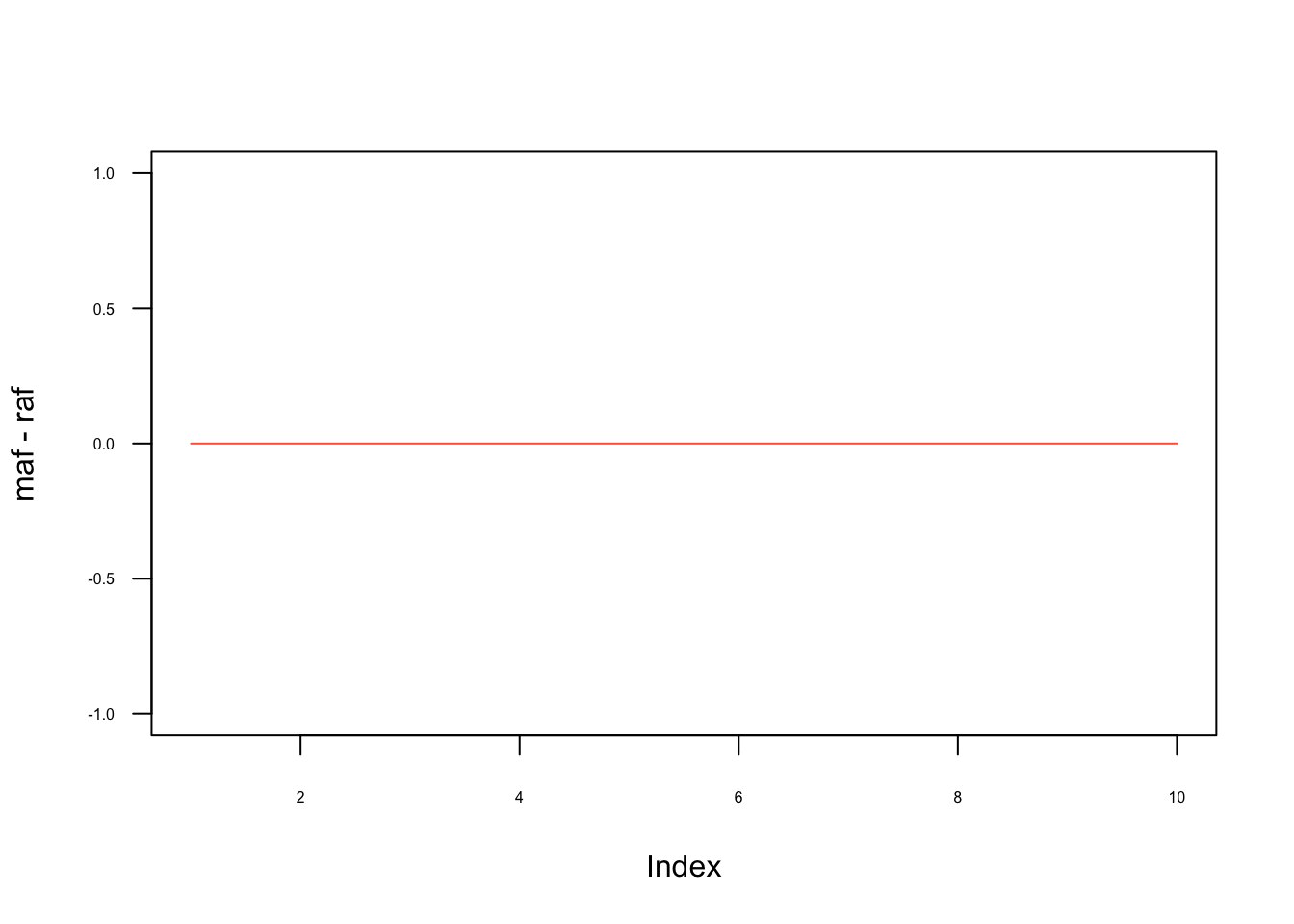## Synopsis

This document documents an example toy analyses of a VCF file with genotyping data using the gwasim package. Package can be installed by typing: devtools::install_github("NBISweden/a_johansson_2020"). For further details visit gwasim GitHub pages.

## Setup

Before starting our simulation, we need to load the necessary packages (install them earlier if you do not already have them in your library). The easiest way is to:

• install.packages('renv'),
• download this Renv lock file,
• renv::restore()

Next, load the packages:

library('vcfR')
library('stringr')
library('gwasim')

## Reading data

For the sake of learning how to use gwasim we will read first 500 rows (markers) from the ALL.chr22.phase3_shapeit2_mvncall_integrated_v5a.20130502.genotypes.vcf.gz file. This file contains 1000 genomes phase 3 data for human chromosome 22. The file can be downloaded from the 1000 Genomes data repository via FTP link.

input_vcf <- '~/Projects/johansson/ALL.chr22.phase3_shapeit2_mvncall_integrated_v5a.20130502.genotypes.vcf.gz'
vcf_data <- vcfR::read.vcfR(file = input_vcf, nrows = 500)
## Scanning file to determine attributes.
## File attributes:
##   meta lines: 252
##   header_line: 253
##   variant count: 500
##   column count: 2513
##
Meta line 252 read in.
## All meta lines processed.
## gt matrix initialized.
## Character matrix gt created.
##   Character matrix gt rows: 500
##   Character matrix gt cols: 2513
##   skip: 0
##   nrows: 500
##   row_num: 0
##
Processed variant: 500
## All variants processed
markers <- vcf_data@fix[,'ID'] # Extract marker names
coords <- vcf_data@fix[, 'POS'] # Extract genomic marker coordinates

We discovered that we need some simple data cleaning because we had few problematic entries in the VCF file. You should always examine your particular dataset for any oddities, try to understand their source and either filter them out or, better, fix them in some other way.

to_remove <- which(str_detect(markers, ";")) # detect weird markers (multi-marker)
markers <- markers[-to_remove]
coords <- coords[-to_remove]

## Get genotypes

Now, let us build a genotyping matrix $$G$$. We will narrow down our scope to the first 10 markers. First, we use gwasim::get_genotypes() to retrieve the genotypes, next we pipe our data stream to the gwasim::fix_allele_encoding function which recodes the $$G$$ matrix. By doing so, we make sure the major homozygote will be encoded as 0, minor homozygote as 2 and heterozygotes as 1. In another words, our genotyping matrix now contains a per locus count of minor alleles. Finally, we impute the missing genotypes by inserting the most common genotype at all NA loci.

Note that this step may potentially change which allele is minor but it will not be reflected in the encoding.

G <- gwasim::get_genotypes(x = vcf_data, marker_names = markers[1:10]) %>%
gwasim::fix_allele_encoding() %>%
gwasim::impute_G()

## Compute maf

Once the data have been loaded, we can start looking at the data. Let’s plot e.g. the minor allele frequency and check that it is the same as the reference (minor) allele frequency, i.e. that the gwasim::fix_allele_encoding function worked as it is supposed to and to see if imputations had any effect on maf.

raf <- colSums(G) / (2 * dim(G))
maf <- pmin(raf, 1 - raf)
plot(maf, cex=.5, col='slateblue', type='l', las=1, cex.axis=.5)plot(maf-raf, cex=.5, col='tomato', type='l', las=1, cex.axis=.5)## Simulate effect of rare alleles for genotype

Now, it is time to do some basic simulations, i.e. to simulate phenotypic values based on the genotypes in our G. To this end, we will use gwasim::get_effects. In this simple example (we will show more complex scenarios later in this document), we would like to simulate the following genetic architecture affecting phenotype:

• there are two randomly selected loci in the region that affect the phenotype,
• loci are selected only from the rare alleles with $$maf <= 0.01$$,
• the effects of the two loci are drawn from Beta distribution with shape parameters set to .1 and .1,
• all effects are positive, i.e. both selected loci have an additive positive effect.
effects <- gwasim::get_effects(maf=maf, thr=0.01, N=2, get_betas_fun = dbeta, get_betas_args = list(shape1 = .1, shape2 = .1), rare=T, frac_negative=0, seed = 2)
effects
## $marker_idx ## rs587769434 rs587638893 ## 5 6 ## ##$effects
## rs587769434 rs587638893
##   108.38947    58.09492

Here, we can see that, indeed, two markers have been selected: rs587720402 and rs587638893 and the effects at these loci are: $$\beta_{rs587720402} = 13.66754$$ and $$\beta_{rs587638893} = 31.14350$$ respectively. We are than ready to simulate our phenotype.

## Compute simulated phenotypes

First, we want some noise in our phenotype to simulate the effect of environment, other loci or simply the measurement error. Let us add some very tiny noise here sampled from $$\mathcal{N}(0, 1)$$.

error_term <- rnorm(n = dim(G), mean = 0, sd = 1)
# Check the error is as you want it
plot(error_term, pch=19, cex=.5, las=1)